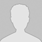# 化学自习室移动版

### 质子守恒三种思维模型

1、质子守恒方法梳理

1.1代数法

1.2框图法

1.3数轴法

2、实例分析

2.1单一溶液 a mol/L NH4HCO3溶液

2.1.1代数推演法：

2.1.2框图法：c(NH3・H2O) ＋ c(CO32) ＋ c(OH) = c(H2CO3) ＋ c(H)

2.1.3数轴解析法：c(NH3・H2O) ＋ c(CO32) ＋ c(OH) = c(H2CO3) ＋ c(H)

2.2不同浓度双组分溶液：a mol/L(NH4)2CO3b mol/L NH4HCO3溶液

2.2.1代数推演法

(2ab)× c(H2CO3) ＋ a×c(HCO3)＋(abc(H)=(ab) ×c(NH3・H2O) ＋ b×c(CO32)＋ (ab)× c(OH)

2.2.2框图法：

(1)组分分离法一:a×c(HCO3) ＋ 2a×c(H2CO3) ＋ a×c(H) = 2a×c(NH3・H2O) ＋ a×c(OH)……①c(H2CO3)＋b×c(H)=b× C(CO32) ＋ b× c(NH3・H2O) ＋ b× c(OH)……②

(2)组分分离法二:

c(NH3・H2O) ＋ c(OH) = c(HCO3) ＋ 2c(H2CO3) ＋ c(H) －b/(ab)× [C(CO32) ＋ c(HCO3) ＋ c(H2CO3)]

(ab)×c(NH3・H2O) ＋ (ab)×c(OH) ＋ b× c(CO32) = a× c(HCO3) ＋ (ab)× c(H) ＋ (2ab)× c(H2CO3)

(3)组分分离法三:c(HCO3) ＋2c(H2CO3) ＋ c(H) －b = c(OH) ＋c(NH3・H2O)……………………①c(H2CO3) ＋ c(H) = c(OH) ＋ c(NH3・H2O) ＋ c(CO32) －a……………………②

①×a ＋ ②×b 得：

(ab)×c(H) ＋ a× c(HCO3) ＋ (2ab)×c(H2CO3) = (ab)×c(OH) ＋(ab)×c(NH3・H2O) ＋ b×c(CO32)

(4)框图统筹法:a/(ab)×c(HCO3)＋2a/(ab)×c(H2CO3) ＋a/(ab)× c(H) = 2a/(2ab)×c(NH3・H2O) ＋a/(ab)×c(OH)b/(ab)×c(H2CO3) ＋ b/(ab)×c(H) = b/(2ab)×c(NH3・H2O) ＋ b/(ab)×c(OH) ＋b/(ab)×c(CO32)

(2ab)×c(H2CO3) ＋ a×c(HCO3) ＋(ab)×c(H) = (ab)×c(NH3・H2O) ＋ (ab)×c(OH) ＋ b×c(CO32)

2.2.3数轴法c(OH)－c(H) = a/(ab)×c(HCO3) ＋ (2ab)/(ab)×c(H2CO3) －b/(ab)×c(CO32) －c(NH3・H2O)

(ab)×c(OH) ＋ b×c(CO32) ＋ (ab)×c(NH3・H2O) =(ab)×c(H) ＋a×c(HCO3) ＋(2ab)×c(H2CO3)

(责任编辑：化学自习室)• 全部评论（0
还没有评论，快来抢沙发吧！
相关文章
作者其他文章
最近关注
热点内容